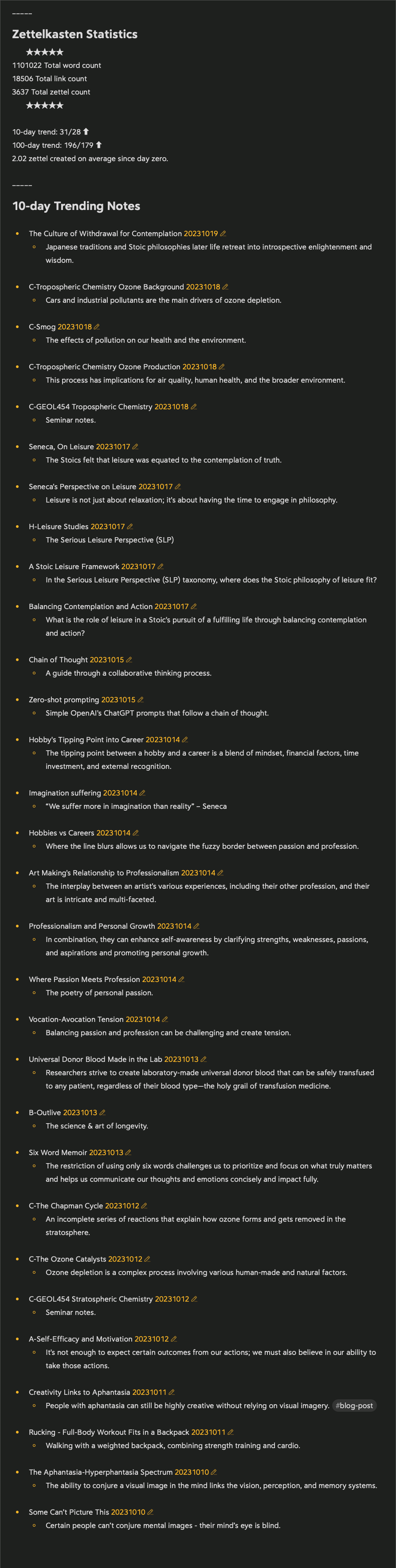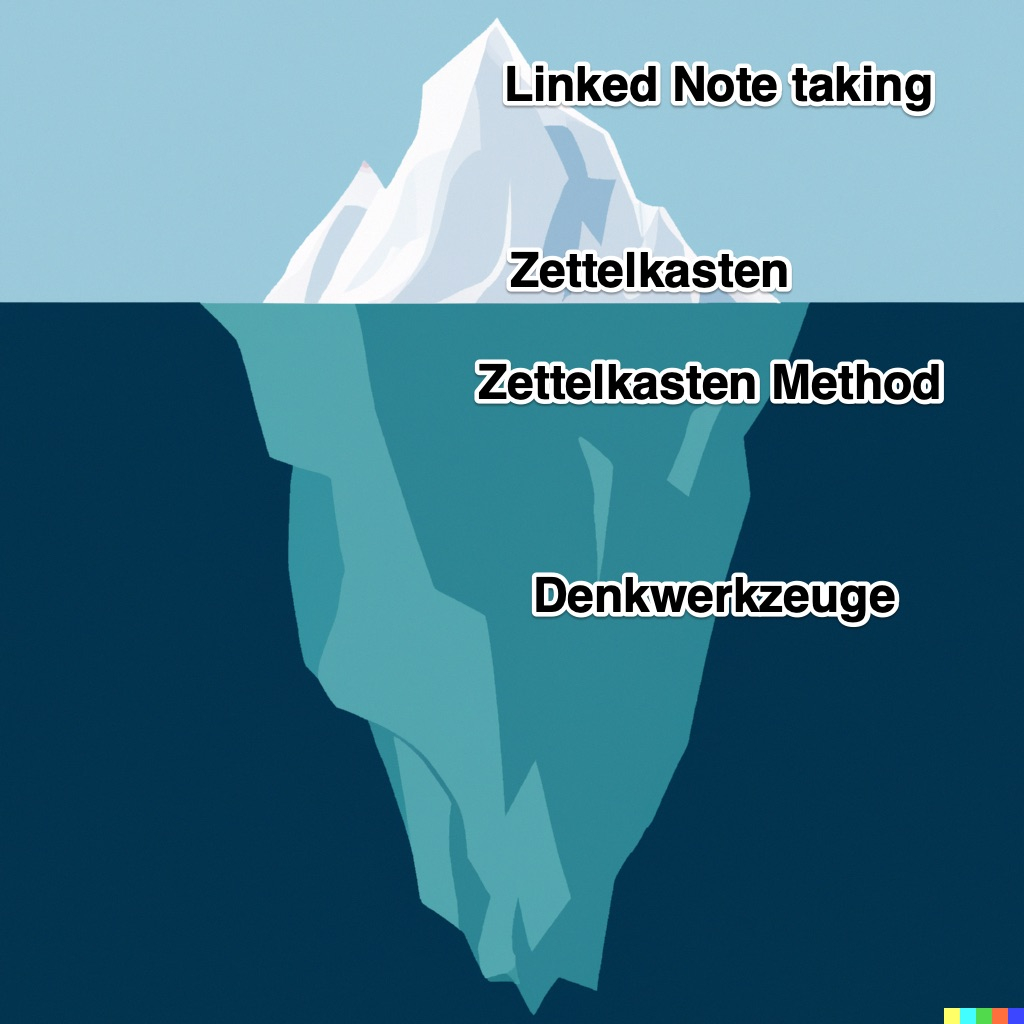# Share with us what is happening in your ZK this week. October 19, 2023

#### Swimming with Ideas

Another installment of the What Are You Working On? thread. Add to this thread by telling us what is happening in and around your ZK journey. Join the community and enlighten us about your knowledge path trajectory. I do this for selfish reasons. It helps me clarify my goals and visualize my thinking. And sometimes, a conversation sparks an idea worth exploring.

#### Ideas I'm exploring with my ZK:

• Atmospheric Chemical Kinetics
• Modern ideas of leisure
• The Stoic idea of leisure
• CoT and Zero-Shot prompting
• Professionalism's relationship to art-making

• Lispector, Clarice. Too much of life: the complete crônicas. 2022.
• Davis, Lydia. Essays one. 2020.
• Chabon, Michael. The Yiddish Policemen's Union. 2008.
• Attia, Peter and Gifford, Bill. Outlive: the science & art of longevity. 2023.

#### Music I'm listening to:

★★★★★

The "My rolling ten-day zettel production" is produced by a script for attachment to my daily journaling template. I do my journaling in Bear to keep personal journaling separate from my knowledge work.

Let me know if you would like to discuss any of these notes.

My ten. day zettelproductionWill Simpson
I must keep doing my best even though I'm a failure. My peak cognition is behind me. One day soon I will read my last book, write my last note, eat my last meal, and kiss my sweetie for the last time.
kestrelcreek.com

• #### Ideas I'm exploring with my ZK:

• The deeper layer of the Zettelkasten Iceberg
• How to learn from God Emperor of Dune how to write believable very old characters (a couple of minutes of free time)
• The problem of reward as a concept (reinforcement should be used most of the time)
• Relapsing (as a more generalised concept)
• The relationship between will and happiness• Stealing Fire by Kotler
• Four Thousand Weeks by Burkemann

#### Music I'm listening to:

• The Witcher 3 Soundtrack
• Some 80s metal with my daughter

★★★★★

*Rolling ten-day zettel production"

202310181145 Ü2 Dopamine fasting
202310181010 Ü2 Reward centre Reinforcer centre
202310180927 Ü2 The relapse
202310180725 Not being able to want makes you unhappy
202310170747 Ü4 The problem with outputs
202310151631 Neurotransmitters and attention
202310151127 Ü2 Leto II Atreides
202310151042 Ü4 How notes help with writer's block
202310111237 Places of worhsip
202310111232 Ü4 Project-specific Zettelkasten
202310111036 PD Workshop Book writing with the Zettelkasten
202310111035 Outline method - focus on the result
202310110701 Ü4 Projects in the Zettelkasten

I am a Zettler

• edited October 23

Notes for a new GitHub repository:

# Opérations Tensorielles Simpliciales

Répertoire pour l'exploration des opérations simpliciales sur les matrices et les hypermatrices.

## Formalités Mathématiques

Ce projet se concentre sur les opérations simpliciales sur les matrices et hypermatrices, en particulier les opérations de face (d_i), de dégénérescence (s_i), et de bord. Les opérations étudiées ici satisfont aux identités simpliciales.

( \begin{aligned} d_i d_j &= d_{j-1} d_i, \quad \text{ si } i < j; \\ s_i s_j &= s_j s_{i-1}, \quad \text{ si } i > j; \\ d_i s_j &= \begin{cases} s_{j-1} d_i, \text{ si } i < j; \\ 1, \qquad \text{ si } i \in \lbrace j, j+1\rbrace; \\ s_j d_{i-1}, \text{ si } i > j+1. \end{cases} \end{aligned} )

The README.md is in French, almost in the style of Cahiers de Topologie et Géométrie Différentielle Catégoriques. As I get further along with this project--the "output" of my Zettelkasten--I'll add to the README in German. The aims and motivation of Opérations Tensorielles Simpliciales, some of which are philosophical, will remain a Pythagorean secret until the applications bear fruit. So far, the algorithms provide concrete representations of $(\infty)$-categories (more precisely, a way to generate them), any one of which can have inner horns of simplices with non-unique fillers in as high a dimension as you might want. Some of the code comments are in French.

While using Moore's algorithm to solve related matrix completion problems, I added a note on an article in Mathematics Magazine.

---
title: "Math.2.1a.0.23.1021 Corrected statement in 'On Reconstructing Matrices'"
reference-section-title: References
---

# Math.2.1a.0.23.1021 Corrected statement in 'On Reconstructing Matrices'

[[Math.2.1.0.23.1021]] Note on a proof from 'On Reconstruction of Matrices'

#matrix #combinatorics #matrix-reconstruction

The proof in (Manvel and Stockmeyer 1971) reconstructs an $(n\times n)$ matrix from a set $(S)$ of its principal submatrices, provided $(n\ge 5)$ and $(S\ge\lceil n/2\rceil +1)$. However, the wording of the proof is misleading. The authors write about the number of times the element $(a_{i, i})$ appears in a principal submatrix. "To reconstruct $(A)$, we first find the diagonal entries by noting that the list of entries $(b_{i,i;k})$ contains $(n-i)$ copies of $(a_{i, i})$ and $(i)$ copies of $(a_{i+1, i+1})$." Here, $(b_{i, i, k})$, is the $(i)$-th diagonal element of $(B_k)$, where $(B_k = A_{\sigma k})$ is a permutation of the ordered list of principal submatrices of $(A)$, with $(A_k)$ being the $(k)$-th principal submatrix for $(1\le k\le n)$. This could be confusing as the element $(a_{i, i})$ occurs in at least $(n-1)$ of the $(n)$ principal submatrices of an $(n\times n)$ matrix. This statement is precise: the element $(a_{i, i})$ appears $(n-i)$ times in the $(i)$-th row and $(i)$-th column of the submatrices (of $(S)$) and the $(i)$-th row and $(i)$-th column of the matrix $(A)$. Also, $(a_{i+1, i+1})$ appears in $(i)$ of the principal submatrices in the same position as in the original matrix, for $(1\le i < n)$.

Perhaps the cost of publication at the time led the authors to sacrifice precision for concision.

## References

Manvel, Bennet, and Paul K. Stockmeyer. 1971. "On Reconstruction of Matrices." Mathematics Magazine 44 (4): 218–21. https://doi.org/10.1080/0025570X.1971.11976153.

Post edited by ZettelDistraction on

GitHub. Erdős #2. CC BY-SA 4.0.

Problems worthy of attack prove their worth by hitting back. -- Piet Hein.